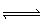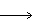Name:    Practice Test 1B

Multiple Choice
Identify the letter of the choice that best completes the statement or answers the question.

1.

Decomposition of NO2 occurs at 25 °C with Kc= 1.83 x 10–14.  What is Kp for this reaction?

2 NO2 (g)N2 (g) + 2 O2 (g)
 A) 3.76 x 10–14 B) 8.92 x 10–15 C) 7.48 x 10–15 D) 4.48 x 10–13

2.

A chemical system at equilibrium will
 A) form more products if temperature is increased. B) have the same concentration of all products and reactants. C) have a specific ratio of products to reactant concentrations. D) not have any precipitates.

3.

For the equlibrium shown below, which change will NOT be effective in increasing the amount of N2O4 (g)?
2 NO2 (g)N2O4 (g)A) adding more NO2 to the reaction vessel B) decreasing the volume of reaction vessel C) removing N2O4 from reaction vessel D) decreasing the temperature

4.

The equilibrium constant (Kc) for the decomposition of COBr2
COBr2 (g)CO (g) + Br2 (g)

is 0.190.  What is Kc for the following reaction?
2 CO (g) + 2 Br2 (g)2 COBr2 (g)
 A) 10.5 B) 2.63 C) 27.7 D) 0.0361

5.

Cyclopropane is converted to propene in a first-order process.  The rate constant is 5.4 x 10–2 hr-1.  If the initial concentration of cyclopropane is 0.150 M, what will its concentration be after 22.0 hours?
 A) 0.105 M B) 0.0457 M C) 0.492 M D) 0.127 M

6.

Given the two equlibria shown below:
Cd2+ (aq) +  S2O32– (aq)CdS2O3 (aq)              Kc= 8.3 x 103
CdS2O3 (aq) +  S2O32– (aq)Cd(S2O3)22– (aq)           Kc= 2.5 x 102
What the value for the equilibrium constant for the following reaction?
Cd2+ (aq) + 2 S2O32– (aq)Cd(S2O3)22– (aq)
 A) 2.1 x 106 B) 8.1 x 103 C) 33 D) 8.6 x 103

7.

A fast reaction should have
 A) a low activation energy B) an exothermic heat of reaction C) a large equilibrium constant D) a catalyst present

8.

The rate law for a particular reaction is found to be

Rate = k[A]2[B]

Which of the following actions will NOT change the initial reaction rate?
 A) doubling the concentration of both A and B B) halving the concentration of A and doubling the concentration of B C) doubling the concentration of A and halving the concentration of B D) halving the concentration of A and quadrupling the concentration of B

9.

For which of the following will Kp = Kc?
 A) 2 NO (g) + O2 (g)N2O4 (g) B) MgCO3 (s) + 2 HCl (g)MgCl2 (s) + CO2 (g) + H2O (l) C) C (s) + O2 (g)CO2 (g) D) Zn (s) + 2 HCl  (aq)ZnCl2 (aq) + H2 (g)

10.

A first order reaction has a half-life of 36 min.  What is the value of rate constant for this reaction?
 A) 1.9 x 10–2 s–1 B) 3.2 x 10–4 s–1 C) 1.2 s–1 D) 0.028 s–1

11.

In the reaction below, the rate of disappearance of H2 is found to be 4.8 x 10–2 M/s.  What is the rate of disappearance of N2 in this reaction?

N2 (g)  +   3 H2 (g)2 NH3 (g)
 A) 1.4 x 10–1 M/s B) 3.2 x 10–2 M/s C) 4.8 x 10–2 M/s D) 1.6 x 10–2 M/s

12.

A mixture of 0.600 mol of bromine and 1.60 mol of iodine is placed in a 1.0-L flask at 350°C.  When the reaction has come to equilibrium, the concentration of IBr is 1.19 M.  What is the equilibrium constant for this reaction?

Br2 (g)  +  I2 (g)2 IBr (g)
 A) 325 B) 3.55 x 10–3 C) 282 D) 1.47

13.

A reaction in which the rate and the rate constant have the same units is a
 A) zero order reaction B) second order reaction C) first order reaction D) radioactive decay

14.

The kinetics of the decomposition of N2O5 is studied at 50°C and 75°C.  Which of the following statements concerning the study is correct?
 A) The rate at 75°C will be greater than the rate at 50°C because the activation energy will be lower at 75°C than at 50°C. B) The rate at 75°C will be greater than the rate at 50°C because the number of molecules with enough energy to react increases with increase in temperature. C) The rate at 75°C will be greater than the rate at 50°C because the concentration of the gas increases with increasing temperature. D) The rate at 75°C will be lower than the rate at 50°C because the molecules at higher speeds do not interact as well as those at lower speeds.

15.

Which of the following CANNOT affect the extent of reaction?
 A) increasing the amount of reactants B) changing the temperature C) changing the volume D) adding a catalyst